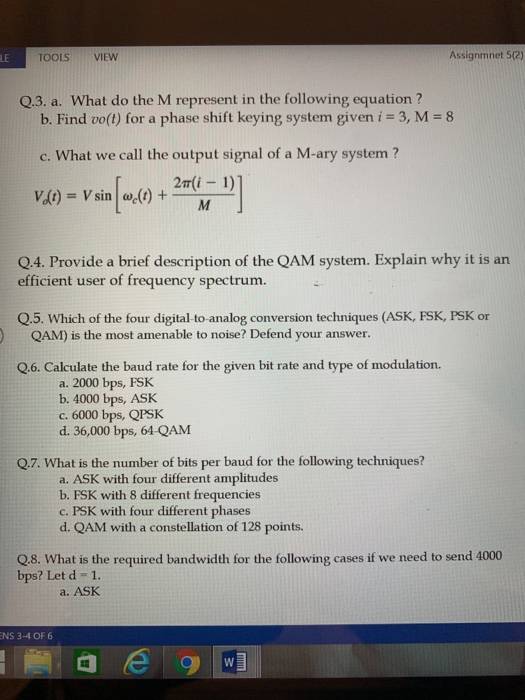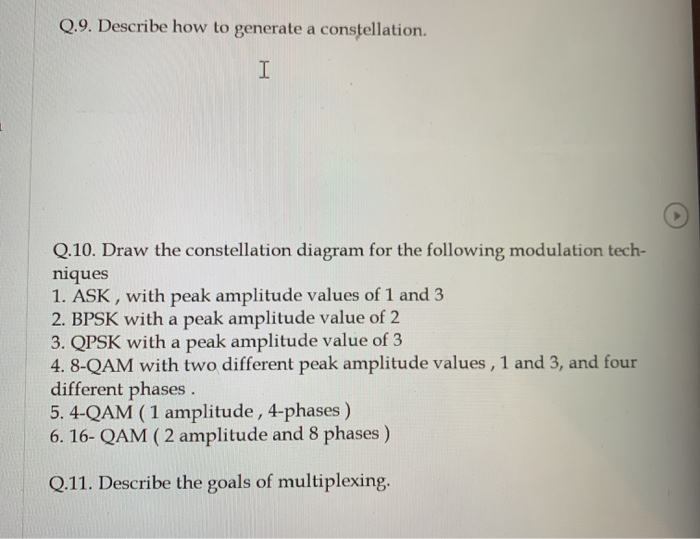### Create an Account

Home / Questions / Assignmnet 5(2) TOOLS VIEW LE Q.3. a. What do the M represent in the following equation? b. Find vo(

# Assignmnet 5(2) TOOLS VIEW LE Q.3. a. What do the M represent in the following equation? b. Find vo(Assignmnet 5(2) TOOLS VIEW LE Q.3. a. What do the M represent in the following equation? b. Find vo(t) for a phase shift keying system given i 3, M 8 c. What we call the output signal of a M-ary system? 2T (i-1) Vlt)= V sin w(t) + Q.4. Provide a brief description of the QAM system. Explain why it is an efficient user of frequency spectrum. Q.5. Which of the four digital-to-analog conversion techniques (ASK, FSK, PSK or QAM) is the most amenable to noise? Defend your answer. Q.6. Calculate the baud rate for the given bit rate and type of modulation. a. 2000 bps, FSK b. 4000 bps, ASK c. 6000 bps, QPSK d. 36,000 bps, 64-QAM Q.7. What is the number of bits per baud for the following techniques? a. ASK with four different amplitudes b. FSK with 8 different frequencies c. PSK with four different phases d. QAM with a constellation of 128 points. Q.8. What is the required bandwidth for the following bps? Let d 1. cases if we need to send 4000 a. ASK NS 3-4 OF 6 W HIE Q.9. Describe how to generate a constellation. I Q.10. Draw the constellation diagram for the following modulation tech- niques 1. ASK, with peak amplitude values of 1 and 3 2. BPSK with a peak amplitude value of 2 3. QPSK with a peak amplitude value of 3 4.8-QAM with two different peak amplitude values, 1 and 3, and four different phases 5. 4-QAM (1 amplitude, 4-phases) 6. 16- QAM (2 amplitude and 8 phases) Q.11. Describe the goals of multiplexing

Apr 01 2020 View more View LessSubscribe To Get Solution